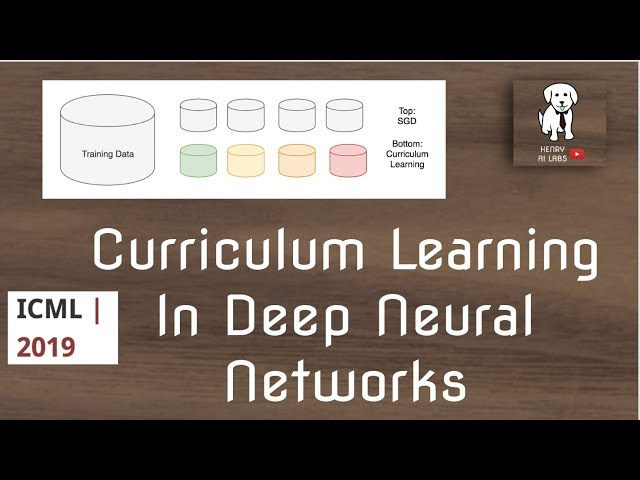# What is Curriculum Learning

##### Curriculum Learning: A Comprehensive Guide

Curriculum learning is a term used in machine learning and deep learning that refers to a technique where the training data is presented to the neural network in a structured manner. The goal of this approach is to improve the performance of the model by presenting the data to the network in a way that maximizes learning efficiency.

This article provides a comprehensive guide to curriculum learning, ranging from its definition, applications, advantages and disadvantages, and some of the popular curriculum learning methods used in deep learning.

##### What is Curriculum Learning?

Curriculum learning is a type of machine learning technique where the training data is presented to the neural network in a structured way. The goal of this approach is to improve the learning efficiency of the model by presenting the data in a way that is optimal for learning.

The name “Curriculum learning” comes from the idea of structuring the training data in a way that mimics the way curricula are structured in schools. In schools, topics are usually presented in a logical order to students, starting from basic concepts to more complex ones. The same principle is used in curriculum learning, where training data is presented to the model in a structured way, starting from easy samples to more difficult ones.

##### Applications of Curriculum Learning

Curriculum learning is used in various applications of machine learning and deep learning, including:

• Image classification
• Natural language processing
• Robotics
• Speech recognition
• Generative models

Curriculum learning has been particularly useful in improving the performance of deep learning models in computer vision tasks, where the learning from easy to difficult examples has been shown to provide better results.

The primary advantage of curriculum learning is its ability to improve the learning efficiency of deep learning models. By presenting the data in a structured way, starting from easy examples to more challenging ones, the model can learn and generalize better. Advantages of curriculum learning include:

• Better performance: Curriculum learning has been shown to improve the performance of deep learning models across various tasks.
• Faster learning: Curriculum learning reduces the time it takes for the model to learn, as it focuses on easy samples first.
• Less overfitting: With curriculum learning, the model is less likely to overfit on the training data, as it is exposed to easier samples first before learning harder ones that have more variation.

Despite its numerous advantages, curriculum learning also has its downsides:

• Increased training time: Curriculum learning usually requires more training time than non-curriculum learning methods.
• Difficulty in selecting the appropriate curriculum: Choosing the appropriate curriculum can be challenging, as it depends on the task and the dataset used.
• May not be suitable for all tasks: Curriculum learning may not be suitable for all tasks, as it largely depends on the structure and nature of the data.
##### Popular Curriculum Learning Methods

There are different curriculum learning methods that are commonly used in deep learning, including:

• Reverse Curriculum Generation
• Dynamic Curriculum Learning
• A Curriculum of Sequences
• Self-Paced Learning
• Teacher-forcing Curriculum Learning

Let's explore them in more detail.

Reverse Curriculum Generation:

In reverse curriculum generation, the training examples are presented to the model in a reverse order, starting from the hardest samples to easier ones. The idea behind this approach is to expose the model to the hardest examples first to make it more robust to variations in the data.

Dynamic Curriculum Learning:

Dynamic curriculum learning is a method where the model gradually increases the difficulty of the examples it is exposed to as it learns. This approach allows the model to learn in a way that is adaptive to its current learning state, as it changes the order of the samples based on the model's performance.

A Curriculum of Sequences:

A Curriculum of Sequences is a method of curriculum learning that is commonly used in language processing tasks. With this approach, the model is not presented with individual samples but with a sequence of samples that follow a logical order. This method allows the model to learn from the context of previous samples and to generate better results.

Self-Paced Learning:

In self-paced learning, the model learns at its own pace by selecting the training examples it wants to learn from. The idea behind this approach is to make the learning process more flexible and adaptive to the model's learning state.

Teacher-forcing Curriculum Learning:

Teacher-forcing curriculum learning is a method where the model is provided with the correct answer for some of the samples it is trained on. This approach allows the model to learn from the correct answers and to improve its performance.

##### The Future of Curriculum Learning

Curriculum learning is a growing field that is expected to play a significant role in improving the performance of deep learning models in the future. As the amount of data used in machine learning tasks continues to grow, curriculum learning is expected to become more critical for enabling models to learn efficiently from large datasets.

Furthermore, curriculum learning is expected to play an essential role in enabling deep learning models to learn from dynamically changing datasets, where some samples may be more relevant to the task than others.

##### Conclusion

Curriculum learning is a valuable technique that can significantly improve the performance, efficiency, and flexibility of deep learning models. It offers numerous advantages over non-curriculum learning, including faster learning, better performance, and less overfitting. While it does have some drawbacks, the benefits of curriculum learning can make it essential to use in many machine learning applications.

As more researchers delve deeper into the potential of curriculum learning, it is likely that this technique will play an increasingly essential role in enabling the next generation of deep learning models to learn more efficiently and effectively from large and complex datasets.